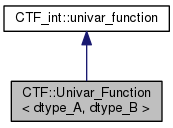Cyclops Tensor Framework parallel arithmetic on multidimensional arrays
CTF::Univar_Function< dtype_A, dtype_B > Class Template Reference

custom function f : X -> Y to be applied to tensor elemetns: e.g. B["ij"] = f(A["ij"]) More...

`#include <functions.h>`

Inheritance diagram for CTF::Univar_Function< dtype_A, dtype_B >:[legend]
Collaboration diagram for CTF::Univar_Function< dtype_A, dtype_B >:[legend]

## Public Member Functions

Univar_Function (std::function< dtype_B(dtype_A)> f_)
constructor takes function pointers to compute B=f(A)); More...

void apply_f (char const *a, char *b) const
apply function f to value stored at a More...

void acc_f (char const *a, char *b, CTF_int::algstrct const *sr_B) const
compute b = b+f(a) More...Public Member Functions inherited from CTF_int::univar_function
virtual bool is_transform () const

univar_function (void(*f_)(char const *, char *))

univar_function (void(*f_)(char const *, char *), bool is_dist)

univar_function ()

univar_function (univar_function const &other)

Unifun_Term operator() (Term const &A) const
evaluate B=f(A) More...

void operator() (Term const &A, Term const &B) const
evaluate B+=f(A) or f(A,B) if transform More...

virtual ~univar_function ()

virtual bool is_accumulator () const

## Data Fields

std::function< dtype_B(dtype_A)> f
function signature for element-wise multiplication, compute b=f(a) More...Data Fields inherited from CTF_int::univar_function
void(* f )(char const *, char *)

bool is_distributive = false

## Detailed Description

### template<typename dtype_A = double, typename dtype_B = dtype_A> class CTF::Univar_Function< dtype_A, dtype_B >

custom function f : X -> Y to be applied to tensor elemetns: e.g. B["ij"] = f(A["ij"])

Definition at line 55 of file functions.h.

## Constructor & Destructor Documentation

template<typename dtype_A = double, typename dtype_B = dtype_A>
 CTF::Univar_Function< dtype_A, dtype_B >::Univar_Function ( std::function< dtype_B(dtype_A)> f_ )
inline

constructor takes function pointers to compute B=f(A));

Parameters
 [in] f_ linear function (type_A)->(type_B)

Definition at line 67 of file functions.h.

## Member Function Documentation

template<typename dtype_A = double, typename dtype_B = dtype_A>
 void CTF::Univar_Function< dtype_A, dtype_B >::acc_f ( char const * a, char * b, CTF_int::algstrct const * sr_B ) const
inlinevirtual

compute b = b+f(a)

Parameters
 [in] a pointer to operand that will be cast to dtype [in,out] b result &f(*a) of applying f on value of (different type) on a [in] sr_B algebraic structure for b, needed to do add

Reimplemented from CTF_int::univar_function.

Definition at line 83 of file functions.h.

References CTF_int::algstrct::add(), and CTF::Endomorphism< dtype >::f.

template<typename dtype_A = double, typename dtype_B = dtype_A>
 void CTF::Univar_Function< dtype_A, dtype_B >::apply_f ( char const * a, char * b ) const
inlinevirtual

apply function f to value stored at a

Parameters
 [in] a pointer to operand that will be cast to dtype [in,out] b result &f(*a) of applying f on value of (different type) on a

Reimplemented from CTF_int::univar_function.

Definition at line 75 of file functions.h.

References CTF::Endomorphism< dtype >::f.

## Field Documentation

template<typename dtype_A = double, typename dtype_B = dtype_A>
 std::function CTF::Univar_Function< dtype_A, dtype_B >::f

function signature for element-wise multiplication, compute b=f(a)

Definition at line 61 of file functions.h.

The documentation for this class was generated from the following file: